﻿ 基于曲面拟合重建单细胞染色体三维结构

# 基于曲面拟合重建单细胞染色体三维结构 Reconstruction of Single-Cell Chromosome

Abstract: Genomics is one of the core areas of bioinformatics; there are two main research directions of genomics, structural genomics targeting whole genome sequencing and functional genomics targeting gene function interpretation. In the past few decades, genomics has experienced considerable development. The prediction of the three-dimensional structure of chromosomes is of great significance for the study of genomics. The reconstruction of the three-dimensional structure of chromosomes is to predict the conformation of the three-dimensional image from the one-dimensional and two-dimensional data of the genome, and then use the data analysis method to judge the reliability of the three-dimensional structure of the reconstructed chromosome. This paper is based on the single-cell chromosome Hi-C technology and Hi-3C derived data to capture the interaction data of individual cells, write the contact frequency matrix, and then convert the contact frequency matrix into a distance matrix to further obtain the three-dimensional structure of the chromosome. The contact matrix of single-cell Hi-C data is sparse and noise-containing, missing many non-contact sites. We refer to such a matrix as a low-rank matrix. The first problem we have to solve is the processing of low rank matrices, also called the completion of low rank distance matrices. This paper introduces several common low-rank matrix completion methods including optimization method and shortest distance method. It also introduces the different methods used in this paper. Finally, the final conclusion is obtained through MATLAB and compared of human research results.

1. 引言

3C实验中产生的接触信息是一个低秩矩阵，染色体的接触频率矩阵是一个0-1矩阵，有接触记为1，无接触记为0。然而0的数目居多，这导致这个接触频率矩阵的秩很低，在转换大距离矩阵时会丢失很多数据。把上述矩阵叫做低秩矩阵。目前，低秩矩阵的完备化一般有两种思路：从优化角度完备低秩矩阵和从数值分析角度完备低秩矩阵。下面分别介绍这两种思路。

2. 常见的缺失数据推断方法

2.1. 利用最优化方法推断缺失数据

$\underset{D\in EDM\left(n\right)}{\mathrm{min}}{‖H\otimes \left(D-\stackrel{˜}{D}\right)‖}_{F}^{2}$ (1)

D中元素个数用d表示，显然d最多等于 $\frac{n\left(n-1\right)}{2}$ ，在大多数应用中，它的秩为 $Ο\left(nr\right)$ ，其中r是最佳嵌入维数，这种度量距离并不是真正意义上的距离，不满足三角不等式。

$\underset{x\ge 0}{\mathrm{min}}{‖H\otimes \left(\kappa \left(x\right)-\stackrel{˜}{D}\right)‖}_{F}^{2}$ (2)

$\kappa \left(x\right)=Diag{1}^{\text{T}}+1Diag{\left(x\right)}^{\text{T}}-2x$$Diag\left(•\right)$ 定义为提取对角线元素，1表示所有和1相等的矢量  。

(2)比(1)有一个实用性的好处，那就是X的秩等于嵌入空间的维数，当矩阵X的秩无约束时，问题(2)是凸的，因此能够解决问题  。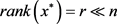(3)

$\underset{x\ge 0}{\mathrm{min}}{‖H\otimes \left(\kappa \left(x\right)-\stackrel{˜}{D}\right)‖}_{F}^{2}$ 使得 $rank\left(x\right)=p$ (4)

$X=Y{Y}^{\text{T}}$ ，其中 $Y\in {R}_{\ast }^{n×p}=\left\{Y\in {R}^{n×p};\mathrm{det}\left(Y{Y}^{\text{T}}\right)\ne 0\right\}$ 为了找到这个矩阵分解，我们采用黎曼流形中的几何框架最优化。参考文献   介绍了矩阵流形最优化更多细节和最新研究成果。

2.2. 利用最短距离法推断缺失数据

Lieberman-Aiden等人研究了两个片段的接触频率、基因线性距离和空间距离之间的关系，表明空间上越接近的片段接触频率值越大，空间距离远的片段产生的接触频率值越小。因此在ShRec3D中有了(5)式的转换函数。Floyd-Warshall算法是一种经典的最短路径算法 ，可以求解图中任意两点之间的最短路径。其主要过程如下：

1) 构建加权图  。假设一个二元组 $G\left(V,E\right)$ 表示一个无向图，其中V表示非空顶点集，每个染色体片段表示图中的顶点；E是无序积V&V的一个多重子集，称E为G的边集，有接触的任意两个片段之间存在一条边，组成边集E  。

2) Floyd-Warshall算法步骤。①令  ，输入权重矩阵 ${D}^{\left(0\right)}={D}^{t}$ 。②令 $k=k+1$ ,计算 ${D}^{\left(k\right)}={\left({D}_{ij}^{\left(k-1\right)}\right)}_{n×n},k=1,2,\cdots ,n$ ，式中 ${D}_{ij}^{k}=\mathrm{min}\left[{D}_{ij}^{\left(k-1\right)},{D}_{ik}^{\left(k-1\right)},{D}_{kj}^{\left(k-1\right)}\right]$ 。③如果k = n，终止算法；否则，返回步骤②。经过这三步，最终结果 ${D}^{\left(n\right)}={\left({D}_{ij}^{\left(n\right)}\right)}_{n×n}$ 中元素 ${D}_{ij}^{n}$ 就是从顶点 ${V}_{i}$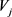的最短路径，从而获得真

3. 利用Hi-C数据得到染色体三维结构

3.1. 将单细胞Hi-C接触矩阵转化为空间距离矩阵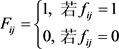(5)

3.2. 通过接触频率矩阵得到欧氏距离矩阵

$F\left(i,j\right)=\frac{|{F}_{i}\cup {F}_{j}|}{|{F}_{i}\cap {F}_{j}|}$ (6)

${y}_{ij}=\frac{{x}_{ij}-\mathrm{min}\left\{{x}_{kl}\right\}}{\mathrm{max}\left\{{x}_{kl}\right\}-\mathrm{min}\left\{{x}_{kl}\right\}}$ (7)

1)主对角线上元素为1；

2)每个元素都在[0, 1]区间内；

$D\left(i,j\right)=\left\{\begin{array}{l}\sqrt{F\left(i,i\right)+F\left(j,j\right)-2F\left(i,j\right)},\text{\hspace{0.17em}}\text{\hspace{0.17em}}\text{if}\text{\hspace{0.17em}}F\left(i,i\right)+F\left(j,j\right)-2F\left(i,j\right)\ge 0\\ 0,\text{\hspace{0.17em}}\text{\hspace{0.17em}}\text{\hspace{0.17em}}\text{\hspace{0.17em}}\text{\hspace{0.17em}}\text{\hspace{0.17em}}\text{\hspace{0.17em}}\text{\hspace{0.17em}}\text{\hspace{0.17em}}\text{\hspace{0.17em}}\text{\hspace{0.17em}}\text{\hspace{0.17em}}\text{\hspace{0.17em}}\text{\hspace{0.17em}}\text{\hspace{0.17em}}\text{\hspace{0.17em}}\text{\hspace{0.17em}}\text{\hspace{0.17em}}\text{\hspace{0.17em}}\text{\hspace{0.17em}}\text{\hspace{0.17em}}\text{\hspace{0.17em}}\text{\hspace{0.17em}}\text{\hspace{0.17em}}\text{\hspace{0.17em}}\text{\hspace{0.17em}}\text{\hspace{0.17em}}\text{\hspace{0.17em}}\text{\hspace{0.17em}}\text{\hspace{0.17em}}\text{\hspace{0.17em}}\text{\hspace{0.17em}}\text{\hspace{0.17em}}\text{\hspace{0.17em}}\text{\hspace{0.17em}}\text{\hspace{0.17em}}\text{\hspace{0.17em}}\text{ }\text{ }\text{\hspace{0.17em}}\text{if}\text{\hspace{0.17em}}F\left(i,i\right)+F\left(j,j\right)-2F\left(i,j\right)<0\end{array}$ (8)

3.3. 利用MDS方法得到染色体三维坐标

MDS  算法的主要步骤如下：

1) 定义一个度量矩阵M，其中M可用距离矩阵D通过(6)式计算得到。

$\begin{array}{l}{d}_{oi}^{2}=\frac{1}{N}\underset{j=1}{\overset{N}{\sum }}{D}_{ij}^{2}-\frac{1}{{N}^{2}}\underset{j=1}{\overset{N}{\sum }}\underset{k>j}{\overset{N}{\sum }}{D}_{jk}^{2}\\ {M}_{ij}=\frac{1}{2}\left[{d}_{oi}^{2}+{d}_{oj}^{2}-{D}_{ij}^{2}\right]\end{array}$ (9)

2) 将M矩阵进行下式的特征值分解。

$M=VA{V}^{\text{T}}$ (10)

$X=V{A}^{\frac{1}{2}}$ (11)

${x}_{i}=\left(\sqrt{{\lambda }_{1}}\ast {\varpi }_{1}\left(i\right),\sqrt{{\lambda }_{2}}\ast {\varpi }_{2}\left(i\right),\sqrt{{\lambda }_{3}}\ast {\varpi }_{3}\left(i\right)\right)$ (12)

4. 结论

4.1. 阐述结论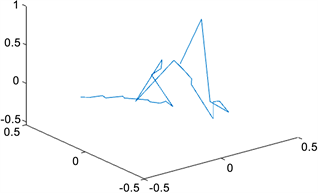Figure 1. Structure of the X chromosome at resolution 6,000,000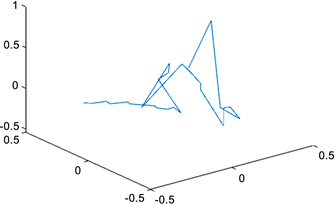Figure 2. Structure of the X chromosome at resolution 8,000,000

4.2. 比较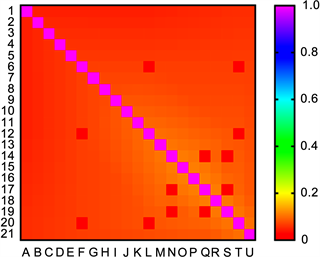Figure 3. Heat map of the contact frequency of each contact site on the X chromosome at a resolution of 8,000,000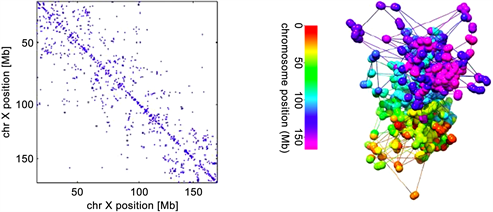Figure 4. Jonas Paulsen uses the optimization toolbox in Matlab to reconstruct the chromosome structure of Manopt

1) 得到了更多的接触频率，使得重构的染色体结构给出染色体非主体部位的结构，弱化了接触位点对于重构后结构的影响，着重描写了染色体的整体结构，即用简单的线条连接每一个接触位点，使每个接触位点都很渺小，从而不影响整体的重构结构；

2) 补充Jonas Paulsen等人的结果中重合部分的结构，如下图所示，Jonas Paulsen等人重构的X染色体3D结构中间部位全是各个接触位点重合的现象，根本没有给出具体的染色体3D结构。这样更有利于人们更好地把握染色体的整体结构。

 彭城, 李国亮, 张红雨, 等. 染色质三维结构重建及其生物学意义[J]. 中国科学: 生命科学, 2014, 44(8): 794-802.

 张卫. 基于Hi-C数据的预测染色体三维结构的方法研究[D]: [硕士学位论文]. 北京: 北京工业大学, 2016.

 Paulsen, J., Gramstad, O. and Collas, P. (2015) Mainifold Based Optimization for Single-Cell 3D Ge-nome Reconstruction. PLoS Computational Biology, 11, e1004396.
https://doi.org/10.1371/journal.pcbi.1004396

 李建更, 张卫, 李晓丹. 基于参数优化的染色体三维结构预测算法VMBO [J]. 北京工业大学学报, 2018, 44(2): 207-214.

 Mishra, B., Meyer, G. and Sepulchre, R. (2011) Low-Rank Optimization for Distance Matrix Completion. 2011 50th IEEE Conference on Decision and Control and European Control Conference, Orlando, FL, 12-15 December 2011, 4455-4460.
https://doi.org/10.1109/CDC.2011.6160810

 Mishra, B. (2014) A Riemannian Geometry for Low-Rank Matrix Completion.

 项荣武, 刘艳杰, 胡忠盛. 图论中最短路径问题的解法[J]. 沈阳航空工业学院学报, 21(2): 86-88.

 Hirata, Y., Oda, A., Ohta, K. and Aihara, K. (2016) Three-Dimensional Reconstruction of Single-Cell Chromosome Structure Using Recurrence Plots. Scientific Reports, 6, Article No. 34982.
https://doi.org/10.1038/srep34982

 Hirata, Y., Horai, S. and Aihara, K. (2008) Reproduction of Distance Ma-trices and Original Time Series from Recurrence Plot and Their Applications. The European Physical Journal Special Topics, 164, 13-22.
https://doi.org/10.1140/epjst/e2008-00830-8

 Tanio, M., Hirata, Y. and Suzuki, H. (2009) Reconstruction of Driving Forces through Recurrence Plots. Physics Letters A, 373, 2031-2040.
https://doi.org/10.1016/j.physleta.2009.03.069

 Varoquaux, N., Ay, F., Noble, W.S. and Vert, J.P. (2014) A Statistical Approach for Inferring the 3D Structure of the Genome. Bioinformatics, 30, i26-i33.
https://doi.org/10.1093/bioinformatics/btu268

 Ben-Elazar, S., Yakhini, Z. and Yanai, I. (2013) Spatial Lo-calization of Co-Regulated Genes Exceeds Genomic Gene Clustering in the Saccharomyces cerevisiae Genome. Nucleic Acids Research, 41, 2191-2201.
https://doi.org/10.1093/nar/gks1360

Top